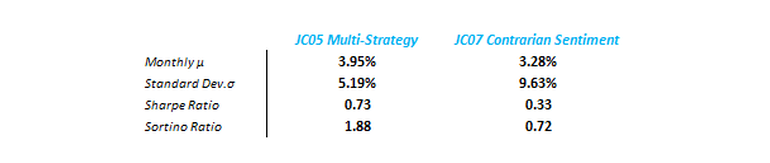Search

# Sortino Ratio – A better way to measure portfolio performance

The foundation of any financial education is built upon two fundamental principles: 1) a dollar is worth more today than tomorrow; Time value of Money. 2) Risk is defined by the uncertainty of an asset’s future value; Volatility. It is from these two fundamental principles that pave the way to all financial and statistical intricacies which mould our understanding and analysis of our prospective investments. However, whilst money does have time value, to say volatility is an appropriate way to measure ‘risk’ is a misnomer.The investment industry standard puts much emphasis on volatility. But what exactly is volatility?

In an investment sense, “Volatility is a statistical measure of the dispersion of returns for a given security or asset. Volatility can either be measured by using the standard deviation (σ) or variance (σ2) between returns from that same security relative to its average or mean (μ)” (‘Volatility’, Investopedia.com). An asset or fund producing returns which disperse vastly around the mean can be said to be more volatile than an asset which produces the same mean, yet its historic returns disperse closer around the same mean. But does more volatility mean greater risk?

Industry standard adopts the Sharpe ratio as a measurement of risk-adjusted return on investments. Average Sharpe ratio for all mid-cap growth funds is approximately 0.30 (“Sharpe Ratio – Morningstar.com”)

Risk is defined by the oxford dictionary as “the chance of bad consequences occurring.” When making a financial analysis, I feel we should treat risk as exactly that… The risk of bad consequences only. Or more to the point, the risk of draw-down! As a trader, I understand riskiness of a trade to be a measure of that which I stand to lose. This is why volatility fails in its depiction of risk.

“Few investors gripe about risk when it turns up handsome gains.” (Frank. A Sortino)

Conventional measures of risk, such as variance and standard deviation punish an investment’s upside potential equally as much as historic losses. Thus a more appropriate measure of risk to be considered is downside risk, or risk of loss.  This can be achieved through analysis of the standard deviation of negative returns of an asset.

The Sortino ratio is “a modification of the Sharpe ratio but uses downside deviation rather than standard deviation as the measure of risk.” (Rollinger, "Sortino: A ‘Sharper’ Ratio - Chicago Mercantile Exchange"). It provides a truer depiction of what an investor stands to lose against the expected returns an investor will make in excess of the risk free rate.

The formula is as follows:

Sortino ratio = (Rp - Rf) / σd

Rp = expected portfolio returns Rf = risk-free interest rate σd = downside deviation of the portfolio

Image 1:  below shows JC07 monthly returns (blue) with mean, Rp-Rf (orange)Consider our two investment strategies…The JC05 Multi-Strategy has half the volatility of the JC07 Contrarian Sentiment Strategy and approximately the same average return. A conventional analysis of the risk-reward trade-off, as measured by the Sharpe ratio using the US 10 year treasury yield, suggests that the JC07 Contrarian Sentiment Strategy is less efficient in its use of volatility to generate profits. The Sortino ratio reveals the JC05 Multi-Strategy to be more efficient in its use of the downside variance to generate profit. This tells us that the profit JC05 generates, relative to what the actually risk of loss an investor faces, is greater than that of the JC07.

However, all ratios, facts, figures and graphs, no matter how intricate, are all merely a snapshot in time as reflected by historic data. All that technical data analysis reveals about an investment is that which has already happened. ‘Past performance is not indicative of future performance,’ however it is all we have.

At times the Sharpe and Sortino ratio portray contrasting preference between investments. This illustrates that risk is a multi-faceted construct which requires deep analysis for truer understanding. Whilst the JC05 Multi-Strategy, by both measures, offers a greater risk-adjusted return, I observe many clients opting for JC07 as for its potential to generate greater absolute return. Investment preference can be scattered across the spectrum of risk-reward utility and  as humans it is our loss aversion which all the more heightens our need to first understand risk before making an investment choice, as we fear that which is averse and unknown. Investors should strive for deeper understanding of risk, as it paves the way to greater utilisation of the risk, and hopefully greater return.

References:

Rollinger, T. N. (n.d.). Sortino: A ‘Sharper’ Ratio - Chicago Mercantile Exchange. Retrieved October 31, 2016, from http://www.cmegroup.com/education/files/sortino-a-sharper-ratio.pdf

Sharpe Ratio. (n.d.). Retrieved October 31, 2016, from http://www.morningstar.com/InvGlossary/sharpe_ratio.aspx